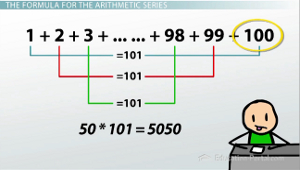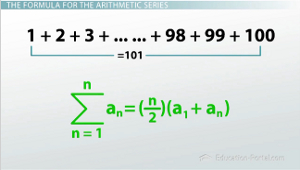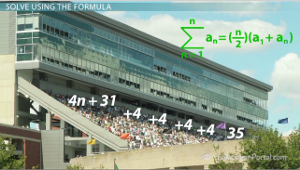# How to Calculate an Arithmetic Series

An error occurred trying to load this video.

Try refreshing the page, or contact customer support.

Coming up next: How to Calculate a Geometric Series

### You're on a roll. Keep up the good work!

Replay
Your next lesson will play in 10 seconds
• 0:02 What is an Arithmetic Series?
• 1:04 The Formula for the…
• 2:51 Solve Using the Formula
• 4:51 Lesson Summary

Want to watch this again later?

Timeline
Autoplay
Autoplay
Speed

#### Recommended Lessons and Courses for You

Lesson Transcript
Instructor: Robert Egan
In this lesson, you'll learn how to sum up any arithmetic sequence without having to add up each and every term. You'll never guess the circumstances that led to the discovery of this marvelous formula.

## What is an Arithmetic Series?An arithmetic sequence is a pattern of numbers that go up by the same amount each time, like 1, 2, 3, 4, 5, … which means that an arithmetic series is what you get when you're asked to add up all the entries within this pattern, like 1+2+3+4+5+…

Well this is exactly what Karl Friedrich Gauss's teacher asked him to do when he was just a boy in elementary school. Apparently, the teacher had had a long day and decided to give the class a little problem that would keep them busy for a while, so that he could maybe take a quick nap.

He instructed them to add up all the numbers between 1 and 100 and then sat down at his desk and put his head down. But much to his dismay, a few minutes later a young Gauss walked up to him and told him the answer, 5050. This must be a joke, thought the teacher, I don't even know the answer to this myself, how could this kid have gotten the answer so fast? It turns out that little Gauss had stumbled upon the formula for any arithmetic series.

## The Formula for any Arithmetic SeriesWhat Gauss noticed is that if he paired up the first and last terms, 1 and 100, he got 101. But if he did the same with the second and second-to-last terms, 2 and 99, he ended up with the exact same thing, 101. If he did the same with 3 and 98, that was still 101. He kept making more and more pairs and noticed that they kept giving him 101. He decided that, since there were 100 terms in the series, there'd be half as many pairs, or 50 pairs of 101. Therefore, he was able to find out that the series 1+2+3+4+….+98+99+100 was just 101x50=5050.

It turns out that this trick will work for any arithmetic series. Simply pair up the first and the last term (a_1 + a_n) and multiply by however many groups you have (n/2), and you get your sum! We therefore have the following formula written in summation notation. We start with the first term and go to n, where n is the number of terms in our series, and we're doing this for some arithmetic sequence a_n. What we get is (n/2), which is the number of terms divided by 2, times the sum of that first and last term (a_1 + a_n). So, that gives us (n/2)(a_1 + a_n), which is our formula for any arithmetic series.

## Solving the Michigan Stadium ProblemTo unlock this lesson you must be a Study.com Member.

### Register to view this lesson

Are you a student or a teacher?

### Unlock Your Education

#### See for yourself why 30 million people use Study.com

##### Become a Study.com member and start learning now.
Back
What teachers are saying about Study.com

### Earning College Credit

Did you know… We have over 160 college courses that prepare you to earn credit by exam that is accepted by over 1,500 colleges and universities. You can test out of the first two years of college and save thousands off your degree. Anyone can earn credit-by-exam regardless of age or education level.